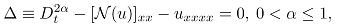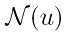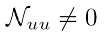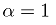# On the Convergence of LHAM and its Application to Fractional Generalised Boussinesq Equations for Closed Form Solutions

• S. O. Ajibola Department of Mathematics, University of Benin, Nigeria
• E. O. Oghre Department of Mathematics, University of Benin, Nigeria
• A. G. Ariwayo Department of Mathematical Sciences, Adekunle Ajasin University, Nigeria
• P. O. Olatunji Department of Mathematical Sciences, Adekunle Ajasin University, Nigeria
Keywords: fractional calculus, Laplace homotopy analysis method, Boussinesq equations, closed form solution

### Abstract

By fractional generalised  Boussinesq equations we mean equations of the formwhereis a differentiable function and(to ensure nonlinearity). In this paper we lay emphasis on the cubic Boussinesq and Boussinesq-like equations of fractional order and we apply the Laplace homotopy analysis method (LHAM) for their rational and solitary wave solutions respectively. It is true that nonlinear fractional differential equations are often difficult to solve for their exact solutions and this single reason has prompted researchers over the years to come up with different methods and approach for their analytic approximate solutions. Most of these methods require huge computations which are sometimes complicated and a very good knowledge of computer aided softwares (CAS) are usually needed. To bridge this gap, we propose a method that  requires no linearization, perturbation or any particularly restrictive assumption that can be easily used to solve strongly nonlinear fractional differential equations by hand and simple computer computations with a very quick run time. For the closed form solution, we setfor each of the solutions and our  results  coincides with those of  others in the literature.

### References

A. Aghili and J. Aghili, Exponential differential operators for singular integral equations and space fractional Fokker-Planck equation, Bol. Soc. Parana. Mat. 36 (2018), 223-233. https://doi.org/10.5269/bspm.v36i1.30959

A. Carpinteri and F. Mainardi, Fractals and Fractional Calculus in Continuum Mechanics, Springer Verlag, Vienna, New York, 1997. https://doi.org/10.1007/978-3-7091-2664-6

A. Shirzadi, L. Ling and S. Abbasbandy, Meshless simulations of the two dimensional fractional-time convection-diffusion-reaction equations, Eng. Anal. Bound. Elem. 38 (2012), 1522-1527. https://doi.org/10.1016/j.enganabound.2012.05.005

A.A. Kilbas, H.M. Srivastava and J.J. Trujillo, Theory and Application of Fractional Differential Equations, Elseiver, 2006.

A.M. Wazwaz, The variational iteration method for rational solutions for KdV, K(2,2), Burgers and cubic Boussinesq equations, Journal of Computational and Applied Math. 207 (2007), 18-23. https://doi.org/10.1016/j.cam.2006.07.010

C.A. Monje, Y. Chen, B.M. Vinagre, D. Xue and V. Feliu-Batile, Fractional-order Systems and Controls: Fundamentals and Applications, Springer-Verlag London Ltd., 2010. https://doi.org/10.1007/978-1-84996-335-0

C. Chen, F. Liu and K. Burrage, Finite difference methods and a Fourier analysis for the fractional reaction-subdiffusion equation, Appl. Math. Comput. 198 (2008), 754-769. https://doi.org/10.1016/j.amc.2007.09.020

D. Kaya, Explicit solutions of generalized Boussinesq equations, J. Appl. Math. 1 (2001), 29-37. https://doi.org/10.1155/S1110757X01000067

D.E. Rupp and J.S. Selker, On the use of the Boussinesq equation for interpreting recession hydrographs from sloping aquifers, Water Resour. Res. 42 (2006), W12421. https://doi.org/10.1029/2006WR005080

E. Ahmed, A.M.A. El-Sayed and H.A.A. El-Saka, Equilibrium points, stability and numerical solutions of fractional-order predator-prey and rabies models, J. Math. Anal. Appl. 325 (2007), 542-553. https://doi.org/10.1016/j.jmaa.2006.01.087

F. Mainardi, Fractional calculus and waves in linear viscoelasticity, An Introduction to Mathematical Models, Imperial College Press, 2010. https://doi.org/10.1142/p614

F. Özpinar, Applying discrete homotopy analysis method for solving fractional partial differential equations, Entropy 20 (2018), 332. https://doi.org/10.3390/e20050332

G. Adomian, A review of the decomposition method in applied mathematics, J. Math. Anal. Appl. 135 (1988), 501-544. https://doi.org/10.1016/0022-247X(88)90170-9

G. Adomian, Solving Frontier Problems in Physics: The Decomposition Method, Kluwer, Boston, 1994. https://doi.org/10.1007/978-94-015-8289-6

G. Wei, J.T. Kirby, S.T. Grilli and R. Subramanya, A fully nonlinear Boussinesq model for surface waves. Part 1. Highly nonlinear unsteady waves, J. Fluid Mech. 294 (1995), 71-92. https://doi.org/10.1017/S0022112095002813

G. Wei and J.T. Kirby, Time-dependent numerical code for extended Boussinesq equations, J. Waterway, Port, Coastal, and Ocean Eng. 121(5) (1995), 251-261. https://doi.org/10.1061/(ASCE)0733-950X(1995)121:5(251)

H. Richard, It's a Nonlinear World, Springer, New York, NY, USA, 2011.

I. Petráš, Fractional derivatives, fractional integrals and fractional differential equations in Matlab, Engineering Education and Research using Matlab, InTech, 2011.

I. Podlubny, Fractional Differential Equations, Academic Press, New York, 1999.

J. Boussinesq, Théorie de l'intumescence appelée onde solitaire ou de translation se propagente dans un canal rectangulaire, Comptes Rendus 72 (1871), 755-759.

J. Boussinesq, Théorie des ondes et des remous qui se propagent le long d'un canal rectangulaire horizontal, en communiquant au liquide contenu dans ce canal des vitesses sensiblemant parielles de la surface au fond, J. Pure Appl. 7 (1872), 55-108.

J. Boussinesq, Essai sur la théorie des eaux courantes, Mem. Acad. Sci. Inst. Fr. 23 (1877), 252-260.

J. Boussinesq, Sur le débit, en temps de sécheresse, d'une source alimentée par une nappe d'eaux d'infiltration, C.R. Hebd. Seanc. Acad. Sci. Paris 136 (1903), 1511-1517.

J. Boussinesq, Recherches théoriques sur l'écoulement des nappes d'eau infiltrées dans le sol et sur débit de sources, J. Math. Pures Appl. 10 (1904), 5-78.

J.A.T. Machado, A probabilistic interpretation of the fractional-order differentiation, Frac. Calc. Appl 6 (2003), 73-80.

J.H. He, Homotopy perturbation technique, Comp. Meth. Appl. Mech. Engnr. 178(3-4) (1999), 256-262. https://doi.org/10.1016/S0045-7825(99)00018-3

J.H. He, Variational iteration method -- a kind of nonlinear analytical technique: some examples, Int. J. Nonlin. Mech. 34(4) (1999), 699-708. https://doi.org/10.1016/S0020-7462(98)00048-1

J.H. He, Variational iteration method. Some recent results and new interpretations, J. Comput. Appl. Math. 207 (2007), 3-17. https://doi.org/10.1016/j.cam.2006.07.009

J.P. Roop, Computational aspects of FEM approximations of fractional advection dispersion equations on bounded domain in R2, J. Comp. Appl. Math. 193 (2006), 243-268. https://doi.org/10.1016/j.cam.2005.06.005

J.S. Russell, Report on waves, Proceedings of the 14th Meeting of the British Association for the Advancement of Science, 1884.

K. Al-Khaled and S. Momani, An approximate solution for a fractional diffusion-wave equation using the decomposition method, Appl. Math. Comp. 165 (2005), 473-483. https://doi.org/10.1016/j.amc.2004.06.026

K. Diethelm, The Analysis of Fractional Differential Equations, Springer, New York, NY, USA, 2010. https://doi.org/10.1007/978-3-642-14574-2

K.B. Oldham and J. Spanier, The Fractional Calculus, Academic Press, New York, USA, 1974.

K.S. Miller and B. Ross, An Introduction to the Fractional Calculus and Fractional Differential Equations, Wiley, New York, 1993.

K.V. Zhukovsky, The operational solution of fractional-order differential equations as well as Black-Scholes and heat-conduction equations, Mosc. Uni. Phys. Bull. 71 (2016), 237-244. https://doi.org/10.3103/S0027134916030164

L. Akinyemi and S.N. Huseen, A powerful approach to study the new modified coupled Korteweg-de Vries system, Math. Comp. Sim. 177 (2020), 556-567. https://doi.org/10.1016/j.matcom.2020.05.021

L. Moslehi and A. Ansari, On M-Wright transforms and time-fractional diffusion equations, Integral transforms Spec. Funct. 28 (2017), 113-129. https://doi.org/10.1080/10652469.2016.1252763

L. Su, W. Wang and Q. Xu, Finite difference methods for fractional dispersion equations, Appl. Math. Comput. 216 (2010), 3329-3334. https://doi.org/10.1016/j.amc.2010.04.060

M. Deghan, J. Manafian and A. Saadatmandi, Solving Nonlinear Fractional Partial Differential Equations using the Homotopy Analysis Method, Wiley InterScience, 2009. https://doi.org/10.1002/num.20460

M. Deghan, M. Abbaszadeh and A. Mohebbi, An implicit RBF meshless approach for solving time fractional nonlinear Sine-Gordon and Klein-Gordon equation, Eng. Anal. Bound. Elem. 50 (2015), 412-434. https://doi.org/10.1016/j.enganabound.2014.09.008

M. Diethelm and A.D. Freed, On the solution of nonlinear fractional order differential equations used in modelling of viscoplasticity, Scientific Computing in Chemical Engineering and Computational Fluid Dynamics, Reaction Engineering and Molecular Properties, Springer, Heidelberg, 1999, pp. 217-224. https://doi.org/10.1007/978-3-642-60185-9_24

M. Eslami, B.F. Vajargah, M. Mirzazadeh and A. Biswas, Applications of first integral method to fractional partial differential equations, Indian J. Phys. 88 (2014), 177-184. https://doi.org/10.1007/s12648-013-0401-6

M. Sajid and T. Hayat, Comparison of HAM and HPM methods in nonlinear heat conduction and convection equations, Nonlinear Anal. Real World Appl. 9 (2008), 2296-2301. https://doi.org/10.1016/j.nonrwa.2007.08.007

M. Zurigat, Solving fractional oscillators using Laplace homotopy analysis method, Annals of the University of Craiova, Mathematics and Computer Science Series 38(4) (2011), 1-11.

M.F. Gobbi, J.T. Kirby and G. Wei, A fully nonlinear Boussinesq model for surface waves. Part 2. Extension to O(kh)4, J. Fluid Mech. 405 (2000), 181-210. https://doi.org/10.1017/S0022112099007247

M.J. Ablowitz, Nonlinear dispersive waves, Asymptotic Analysis and Solitons, Cambridge Univ. Press, 2011.

M.S.H. Chowdhury, I. Hashim and O. Abdulaziz, Comparison of homotopy analysis method and homotopy perturbation method for purely nonlinear fin-type problems, Commun. Nonlinear Sci. Numeri. Simul. 14 (2009), 371-378. https://doi.org/10.1016/j.cnsns.2007.09.005

N. Abel, Solution de quelques problémes á laide dintégrales définites, Christiania Grondhal, Norway, 1881, pp. 16-18.

N.J. Ford and A.C. Simpson, Numerical approaches to the solution of some fractional differential equations, Numerical Analysis Report. Manchester Centre for Computational Mathematics, Manchester, 2003.

N.J. Ford and A.C. Simpson, The numerical solution of fractional differential equations, Numerical Analysis Report, Manchester Centre for Computational Mathematics, Manchester, 2003.

N.T. Shawagfeh, Analytical approximate solutions for nonlinear fractional differential equations, Appl. Math. Comput. 131(2) (2002), 517-529. https://doi.org/10.1016/S0096-3003(01)00167-9

O. Nwogu, An alternative form of the Boussinesq equations for nearoffshore wave propagation, J. Waterway. Port. Coast. Ocean Eng. 119 (1993), 618-638. https://doi.org/10.1061/(ASCE)0733-950X(1993)119:6(618)

P. A. Williams, Unifying Fractional Calculus with Time Scales, PhD thesis, University of Melbourne, 2012.

P. Rosenau and J.M. Hyman, Compactons: Solitons with finite wavelengths, Physical Reviews Letters, American Physical Society 70 (1993), 564-567. https://doi.org/10.1103/PhysRevLett.70.564

P. Rosenau, ''What is a compacton?'', Notices of the American Mathematical Society (2005), 738-739.

R. Abazari and A. Kiliçman, Solitary wave solutions of the Boussinesq equation and its improved form, Math. Probl. Eng., vol. 2013, Article ID 468206. https://doi.org/10.1155/2013/468206

R. Caponetto, G. Dongola, L. Fortuna and I. Petráš, Fractional Order Systems: Modelling and Control Applications, World Scientific, River Edge, NJ, 2010. https://doi.org/10.1142/7709

R. Sahadevan and T. Bakkyaraj, Invariant analysis of time fractional generalized Burgers and Korteweg-de Vries equations, J. Math. Anal. Appl. 2 (2012), 341-347. https://doi.org/10.1016/j.jmaa.2012.04.006

R.L. Magin, Fractional Calculus in Bioengineering, Begell House, 2006.

S. Lacroix, Traité du calcul differentiel et du calcul intégral, Courcier, Paris.

S. Momani and Z. Odibat, Homotopy perturbation method for nonlinear partial differential equations of fractional order, Physics Letters A 365(5) (2007), 345-350. https://doi.org/10.1016/j.physleta.2007.01.046

S.J Liao, The proposed homotopy analysis technique for the solution of nonlinear problems, PhD thesis, Shanghai Jiao Tong University, 1992.

S.O. Ajibola, A.S. Oke and W.N. Mutuku, LHAM approach to fractional order Rosenau-Hyman and Burgers' equations, Asian Research Journal of Mathematics 16(6) (2020), 1-14. https://doi.org/10.9734/arjom/2020/v16i630192

S.S. Ray and R.K. Bera, An approximate solution of a nonlinear fractional differential equation by Adomian decomposition method, Appl. Math. Comp. 167 (2005), 561-571. https://doi.org/10.1016/j.amc.2004.07.020

S.T. Momani and N.T. Shawagfeh, Decomposition method for solving fractional Riccati differential equations, Appl. Math. Comput. 182 (2006), 1083-1092. https://doi.org/10.1016/j.amc.2006.05.008

T. Hayat, S. Nadeem and S. Asghar, Periodic unidirectional flows of a viscoelastic fluid with the fractional Maxwell model, Appl. Math. Comput. 151 (2004), 153-161. https://doi.org/10.1016/S0096-3003(03)00329-1

T.J. Priestly and P.A. Clarkson, Symmetries of a generalized Boussinesq equation, IMS Technical Report, UKC/IMS/59, 1996.

V. Daftardar-Gejji and H. Jafari, Adomian decomposition: A tool for solving a system of fractional differential equations, J. Math. Anal. Appl. 301(2) (2005), 508-518. https://doi.org/10.1016/j.jmaa.2004.07.039

W. Deng, Finite element method for the space and time fractional Fokker-Planck equation, SIAM Journal on Numerical Analysis 47 (2008), 204-226. https://doi.org/10.1137/080714130

Y. Lin and C. Xu, Finite difference/spectral approximations for the time fractional diffusion equation, J. Comput. Phys. 225(2) (2007), 1533-1552. https://doi.org/10.1016/j.jcp.2007.02.001

Y. Pandir and H. Huseyin Duzgun, New exact solutions of time fractional Gardner equation by using new version of F-Expansion method, Commun. Theor. Phys. 67 (2017), 9-14. https://doi.org/10.1088/0253-6102/67/1/9

Y. Zhu, Q. Chang and S. Wu, Exact solitary solutions with compact support for the nonlinear dispersive Boussinesq-like B(m,n) equations, Chaos Solitons and Fractals 26 (2005), 407-413. https://doi.org/10.1016/j.chaos.2005.01.005

Z. Odibat and S. Momani, Application of variation iteration method to nonlinear differential equations of fractional order, Int. J. Nonlin. Sci. Numer. Simul. 1(7) (2006), 15-27. https://doi.org/10.1515/IJNSNS.2006.7.1.27

Published
2021-05-22
How to Cite
Ajibola, S. O., Oghre, E. O., Ariwayo, A. G., & Olatunji, P. O. (2021). On the Convergence of LHAM and its Application to Fractional Generalised Boussinesq Equations for Closed Form Solutions. Earthline Journal of Mathematical Sciences, 7(1), 25-47. https://doi.org/10.34198/ejms.7121.2547
Issue
Section
Articles